# 轻量函数式 JavaScript：八、列表操作

+关注继续查看

## 非 FP 列表处理

• forEach(..)
• some(..)
• every(..)

forEach(..) 是一个迭代帮助函数，但它被设计为对每一个执行的函数调用都带有副作用；你可能猜到了为什么我们的讨论不赞同它是一个 FP 列表操作。

some(..)every(..) 确实鼓励使用纯函数（具体地讲，是像 filter(..) 这样的检测函数），但它们实质上像一个检索或匹配一样，不可避免地将一个列表递减为一个 true / false 的结果。这两个工具不是很适合作为我们想要对代码进行 FP 建模的模具，所以这里我们跳过它们不讲。

## Map

var x = 2, y;

// 变形/投射
y = x * 3;

// 改变/重新赋值
x = x * 3;

var multipleBy3 = v => v * 3;

var x = 2, y;

// 变形/投射
y = multiplyBy3( x );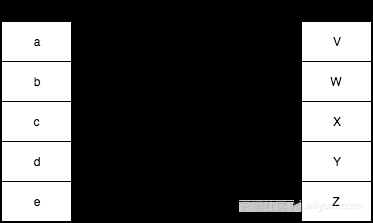function map(mapperFn,arr) {
var newList = [];

for (let idx = 0; i < arr.length; i++) {
newList.push(
mapperFn( arr[i], idx, arr )
);
}

return newList;
}

mapperFn(..) 自然地将项目列表传递给映射/变形，同时还有 idxarr。我们这样做是为了与内建的 map(..) 保持一致。在某些情况下这些额外的信息可能十分有用。

map( ["1","2","3"], unary( parseInt ) );
// [1,2,3]

JavaScript 在数组上提供了内建的 map(..) 工具，使得它很容易地用做一个列表操作链条上的一部分。

var one = () => 1;
var two = () => 2;
var three = () => 3;

[one,two,three].map( fn => fn() );
// [1,2,3]

var increment = v => ++v;
var decrement = v => --v;
var square = v => v * v;

var double = v => v * 2;

[increment,decrement,square]
.map( fn => compose( fn, double ) )
.map( fn => fn( 3 ) );
// [7,5,36]

### 同步 vs 异步

var newArr = arr.map();

arr.addEventListener( "value", multiplyBy3 );

### 映射 vs 迭代

[1,2,3,4,5]
.map( function mapperFn(v){
console.log( v );            // 副作用！
return v;
} )
..

### 一个词：函子

function uppercaseLetter(c) {
var code = c.charCodeAt( 0 );

// 小写字符？
if (code >= 97 && code <= 122) {
// 将它大写！
code = code - 32;
}

return String.fromCharCode( code );
}

function stringMap(mapperFn,str) {
return [...str].map( mapperFn ).join( "" );
}

stringMap( uppercaseLetter, "Hello World!" );
// HELLO WORLD!

stringMap(..) 允许一个字符串是一个函子。你可以为任意数据结构定义一个映射函数；只要这个工具符合这些规则，那么这种数据结构就是一个函子。

## Filter

filter(..) 列表操作用一个函数来决定原来数组中的每一个值是否应该保留在新的数组中。如果一个值应当被保留，那么这个函数需要返回 true，如果它应当被跳过则返回 false。一个为做决定而返回 true / false 的函数有一个特殊的名字：判定函数。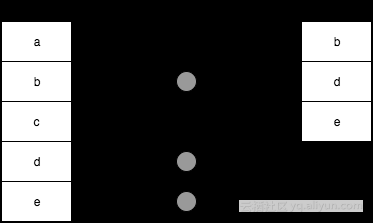function filter(predicateFn,arr) {
var newList = [];

for (let idx = 0; idx < arr.length; idx++) {
if (predicateFn( arr[idx], idx, arr )) {
newList.push( arr[idx] );
}
}

return newList;
}

map(..) 一样，filter(..) 作为一种 JS 数组上的内建工具被提供。

var whatToCallIt = v => v % 2 == 1;

var isOdd = v => v % 2 == 1;

var midIdx;

if (isOdd( list.length )) {
midIdx = (list.length + 1) / 2;
}
else {
midIdx = list.length / 2;
}

[1,2,3,4,5].filter( isOdd );
// [1,3,5]

var isEven = v => v % 2 == 1;

[1,2,3,4,5].filter( isEven );
// [1,3,5]

isEven( 2 );        // false

var isEven = not( isOdd );

isEven( 2 );        // true

[1,2,3,4,5].filter( not( isEven ) );
// [1,3,5]

### 滤除与滤入

var filterIn = filter;

function filterOut(predicateFn,arr) {
return filterIn( not( predicateFn ), arr );
}

isOdd( 3 );                                // true
isEven( 2 );                            // true

filterIn( isOdd, [1,2,3,4,5] );            // [1,3,5]
filterOut( isEven, [1,2,3,4,5] );        // [1,3,5]

## Reduce

map(..)filter(..) 产生一个新的列表，但这第三种操作（reduce(..)）经常将一个列表的值结合（也就是“递减”）为一个单独的有限（非列表）值，比如数字或字符串。但是在本章稍后，我们将会看到如何以更高级的方式使用 reduce(..)reduce(..) 是最重要的 FP 工具之一；它就像瑞士军刀一样多才多艺。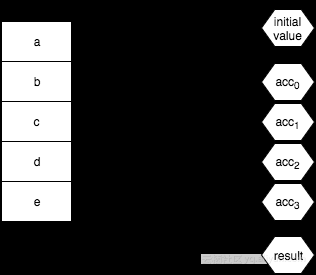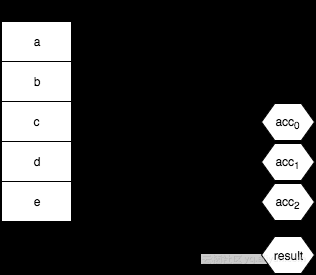1. 3 * 5 = 15
2. 15 * 10 = 150
3. 150 * 15 = 2250

[5,10,15].reduce( (product,v) => product * v, 3 );
// 2250

function reduce(reducerFn,initialValue,arr) {
var acc, startIdx;

if (arguments.length == 3) {
acc = initialValue;
startIdx = 0;
}
else if (arr.length > 0) {
acc = arr;
startIdx = 1;
}
else {
throw new Error( "Must provide at least one value." );
}

for (let idx = startIdx; idx < arr.length; idx++) {
acc = reducerFn( acc, arr[idx], idx, arr );
}

return acc;
}

map(..)filter(..) 一样，递减函数也会被传入不太常用的 idxarr 参数以备递减中的不时之需。我不常用这些东西，但我猜它们可以随时取用是一件不错的事情。

function compose(...fns) {
return function composed(result){
return fns.reverse().reduce( function reducer(result,fn){
return fn( result );
}, result );
};
}

var pipeReducer = (composedFn,fn) => pipe( composedFn, fn );

var fn =
[3,17,6,4]
.map( v => n => v * n )
.reduce( pipeReducer );

fn( 9 );            // 11016  (9 * 3 * 17 * 6 * 4)
fn( 10 );            // 12240  (10 * 3 * 17 * 6 * 4)

var binary =
fn =>
(arg1,arg2) =>
fn( arg1, arg2 );

var pipeReducer = binary( pipe );

var fn =
[3,17,6,4]
.map( v => n => v * n )
.reduce( pipeReducer );

fn( 9 );            // 11016  (9 * 3 * 17 * 6 * 4)
fn( 10 );            // 12240  (10 * 3 * 17 * 6 * 4)

map(..)filter(..) 来说遍历数组的顺序实际上不重要，与此不同的是，reduce(..) 绝对是按照从左到右的顺序处理。如果你想要从右到左地递减，JavaScript 提供了一个 reduceRight(..)，它除了顺序以外其他一切行为都与 reduce(..) 相同：

var hyphenate = (str,char) => str + "-" + char;

["a","b","c"].reduce( hyphenate );
// "a-b-c"

["a","b","c"].reduceRight( hyphenate );
// "c-b-a"

reduce(..) 从左到右地工作，因此在组合函数时很自然地像 pipe(..) 一样动作，而 reduceRight(..) 从右到左的顺序对于 compose(..) 一样的操作来说更自然。那么，让我们使用 reduceRight(..) 来重温 compose(..)

function compose(...fns) {
return function composed(result){
return fns.reduceRight( function reducer(result,fn){
return fn( result );
}, result );
};
}

### Map 作为 Reduce

map(..) 操作天生就是迭代性的，所以它也可以表达为一种递减（reduce(..)）。这其中的技巧是要理解 reduce(..)initialValue 本身可以是一个（空）数组，这样递减的结果就可以是另一个列表！

var double = v => v * 2;

[1,2,3,4,5].map( double );
// [2,4,6,8,10]

[1,2,3,4,5].reduce(
(list,v) => (
list.push( double( v ) ),
list
), []
);
// [2,4,6,8,10]

### Filter 作为 Reduce

var isOdd = v => v % 2 == 1;

[1,2,3,4,5].filter( isOdd );
// [1,3,5]

[1,2,3,4,5].reduce(
(list,v) => (
isOdd( v ) ? list.push( v ) : undefined,
list
), []
);
// [1,3,5]

## 高级列表操作

### Unique

var unique =
arr =>
arr.filter(
(v,idx) =>
arr.indexOf( v ) == idx
);

var unique =
arr =>
arr.reduce(
(list,v) =>
list.indexOf( v ) == -1 ?
( list.push( v ), list ) : list
, [] );

unique(..) 可以出色地产生一个没有重复的新列表：

unique( [1,4,7,1,3,1,7,9,2,6,4,0,5,3] );
// [1, 4, 7, 3, 9, 2, 6, 0, 5]

### Flatten

[ [1, 2, 3], 4, 5, [6, [7, 8]] ]

[ 1, 2, 3, 4, 5, 6, 7, 8 ]

var flatten =
arr =>
arr.reduce(
(list,v) =>
list.concat( Array.isArray( v ) ? flatten( v ) : v )
, [] );

flatten( [[0,1],2,3,[4,[5,6,7],[8,[9,[10,[11,12],13]]]]] );
// [0,1,2,3,4,5,6,7,8,9,10,11,12,13]

var flatten =
(arr,depth = Infinity) =>
arr.reduce(
(list,v) =>
list.concat(
depth > 0 ?
(depth > 1 && Array.isArray( v ) ?
flatten( v, depth - 1 ) :
v
) :
[v]
)
, [] );

flatten( [[0,1],2,3,[4,[5,6,7],[8,[9,[10,[11,12],13]]]]], 0 );
// [[0,1],2,3,[4,[5,6,7],[8,[9,[10,[11,12],13]]]]]

flatten( [[0,1],2,3,[4,[5,6,7],[8,[9,[10,[11,12],13]]]]], 1 );
// [0,1,2,3,4,[5,6,7],[8,[9,[10,[11,12],13]]]]

flatten( [[0,1],2,3,[4,[5,6,7],[8,[9,[10,[11,12],13]]]]], 2 );
// [0,1,2,3,4,5,6,7,8,[9,[10,[11,12],13]]]

flatten( [[0,1],2,3,[4,[5,6,7],[8,[9,[10,[11,12],13]]]]], 3 );
// [0,1,2,3,4,5,6,7,8,9,[10,[11,12],13]]

flatten( [[0,1],2,3,[4,[5,6,7],[8,[9,[10,[11,12],13]]]]], 4 );
// [0,1,2,3,4,5,6,7,8,9,10,[11,12],13]

flatten( [[0,1],2,3,[4,[5,6,7],[8,[9,[10,[11,12],13]]]]], 5 );
// [0,1,2,3,4,5,6,7,8,9,10,11,12,13]

#### Map，之后 Flatten

flatten(..) 行为的最常见用法之一是在你映射一个列表时，每一个从原数组变形而来的元素本身就是一个值的列表。例如：

var firstNames = [
{ name: "Jonathan", variations: [ "John", "Jon", "Jonny" ] },
{ name: "Stephanie", variations: [ "Steph", "Stephy" ] },
{ name: "Frederick", variations: [ "Fred", "Freddy" ] }
];

firstNames
.map( entry => [entry.name].concat( entry.variations ) );
// [ ["Jonathan","John","Jon","Jonny"], ["Stephanie","Steph","Stephy"],
//   ["Frederick","Fred","Freddy"] ]

flatten(
firstNames
.map( entry => [entry.name].concat( entry.variations ) )
);
// ["Jonathan","John","Jon","Jonny","Stephanie","Steph","Stephy","Frederick",
//  "Fred","Freddy"]

FP 库中经常定义一个“映射之后平整”组合的 flatMap(..)（也常被称为 chain(..)）。为了一致性和易于（通过柯里化）组合，这些 flatMap(..) / chain(..) 工具通常都与我们以前看到的 map(..)filter(..)、和 reduce(..) 独立工具的 mapperFn, arr 参数顺序相吻合。

flatMap( entry => [entry.name].concat( entry.variations ), firstNames );
// ["Jonathan","John","Jon","Jonny","Stephanie","Steph","Stephy","Frederick",
//  "Fred","Freddy"]

var flatMap =
(mapperFn,arr) =>
flatten( arr.map( mapperFn ), 1 );

var flatMap =
(mapperFn,arr) =>
arr.reduce(
(list,v) =>
list.concat( mapperFn( v ) )
, [] );

### Zip

zip( [1,3,5,7,9], [2,4,6,8,10] );
// [ [1,2], [3,4], [5,6], [7,8], [9,10] ]

12 被选入子列表 [1,2]，然后 34 被选入 [3,4]，等等。zip(..) 的定义要求从两个列表中各取一个值。如果这两个列表长度不同，那么值的选择将会进行到较短的那个列表耗尽为止，在另一个列表中额外的值将会被忽略。

function zip(arr1,arr2) {
var zipped = [];
arr1 = arr1.slice();
arr2 = arr2.slice();

while (arr1.length > 0 && arr2.length > 0) {
zipped.push( [ arr1.shift(), arr2.shift() ] );
}

return zipped;
}

### Merge

mergeLists( [1,3,5,7,9], [2,4,6,8,10] );
// [1,2,3,4,5,6,7,8,9,10]

zip( [1,3,5,7,9], [2,4,6,8,10] );
// [ [1,2], [3,4], [5,6], [7,8], [9,10] ]

flatten( [ [1,2], [3,4], [5,6], [7,8], [9,10] ] );
// [1,2,3,4,5,6,7,8,9,10]

// 组合后：
flatten( zip( [1,3,5,7,9], [2,4,6,8,10] ) );
// [1,2,3,4,5,6,7,8,9,10]

function mergeLists(arr1,arr2) {
var merged = [];
arr1 = arr1.slice();
arr2 = arr2.slice();

while (arr1.length > 0 || arr2.length > 0) {
if (arr1.length > 0) {
merged.push( arr1.shift() );
}
if (arr2.length > 0) {
merged.push( arr2.shift() );
}
}

return merged;
}

// 由 @rwaldron 编写
var mergeReducer =
(merged,v,idx) =>
(merged.splice( idx * 2, 0, v ), merged);

// 由 @WebReflection 编写
var mergeReducer =
(merged,v,idx) =>
merged
.slice( 0, idx * 2 )
.concat( v, merged.slice( idx * 2 ) );

[1,3,5,7,9]
.reduce( mergeReducer, [2,4,6,8,10] );
// [1,2,3,4,5,6,7,8,9,10]

## 方法 vs. 独立函数

[1,2,3,4,5]
.filter( isOdd )
.map( double )
.reduce( sum, 0 );                    // 18

// vs.

reduce(
map(
filter( [1,2,3,4,5], isOdd ),
double
),
sum,
0
);                                    // 18

### 组合方法链

var partialThis =
(fn,...presetArgs) =>
// 有意地制造一个允许 this 绑定的 function
function partiallyApplied(...laterArgs){
return fn.apply( this, [...presetArgs, ...laterArgs] );
};

var composeChainedMethods =
(...fns) =>
result =>
fns.reduceRight(
(result,fn) =>
fn.call( result )
, result
);

composeChainedMethods(
partialThis( Array.prototype.reduce, sum, 0 ),
partialThis( Array.prototype.map, double ),
partialThis( Array.prototype.filter, isOdd )
)
( [1,2,3,4,5] );                    // 18

### 组合独立函数工具

var filter = (arr,predicateFn) => arr.filter( predicateFn );

var map = (arr,mapperFn) => arr.map( mapperFn );

var reduce = (arr,reducerFn,initialValue) =>
arr.reduce( reducerFn, initialValue );

compose(
partialRight( reduce, sum, 0 )
partialRight( map, double )
partialRight( filter, isOdd )
)
( [1,2,3,4,5] );                    // 18

var filter = curry(
(predicateFn,arr) =>
arr.filter( predicateFn )
);

var map = curry(
(mapperFn,arr) =>
arr.map( mapperFn )
);

var reduce = curry(
(reducerFn,initialValue,arr) =>
arr.reduce( reducerFn, initialValue );

compose(
reduce( sum )( 0 ),
map( double ),
filter( isOdd )
)
( [1,2,3,4,5] );                    // 18

### 将方法适配为独立函数

var unboundMethod =
(methodName,argCount = 2) =>
curry(
(...args) => {
var obj = args.pop();
return obj[methodName]( ...args );
},
argCount
);

var filter = unboundMethod( "filter", 2 );
var map = unboundMethod( "map", 2 );
var reduce = unboundMethod( "reduce", 3 );

compose(
reduce( sum )( 0 ),
map( double ),
filter( isOdd )
)
( [1,2,3,4,5] );                    // 18

### 将独立函数适配为方法

1. 使用额外的方法扩展内建的 Array.prototype
2. 适配一个用于递减函数的独立工具，并将它传入 reduce(..) 实例方法。

var flatten =
arr =>
arr.reduce(
(list,v) =>
list.concat( Array.isArray( v ) ? flatten( v ) : v )
, [] );

// 有意地定义一个允许通过名称进行递归的函数
function flattenReducer(list,v) {
return list.concat(
Array.isArray( v ) ? v.reduce( flattenReducer, [] ) : v
);
}

[ [1, 2, 3], 4, 5, [6, [7, 8]] ]
.reduce( flattenReducer, [] )
// ..

## 寻找列表

var getSessionId = partial( prop, "sessId" );
var getUserId = partial( prop, "uId" );

var session, sessionId, user, userId, orders;

session = getCurrentSession();
if (session != null) sessionId = getSessionId( sessionId );
if (sessionId != null) user = lookupUser( sessionId );
if (user != null) userId = getUserId( user );
if (userId != null) orders = lookupOrders( userId );
if (orders != null) processOrders( orders );

var guard =
fn =>
arg =>
arg != null ? fn( arg ) : arg;

[ getSessionId, lookupUser, getUserId, lookupOrders, processOrders ]
.map( guard )

.reduce(
(result,nextFn) => nextFn( result )
, getCurrentSession()
)

[ "sessId", "uId" ].map( propName => partial( prop, propName ) )

var mergeReducer =
(merged,v,idx) =>
(merged.splice( idx * 2, 0, v ), merged);

.reduce( mergeReducer, [ lookupUser ] )

.concat( lookupOrders, processOrders )

[ "sessId", "uId" ].map( propName => partial( prop, propName ) )
.reduce( mergeReducer, [ lookupUser ] )
.concat( lookupOrders, processOrders )

[ "sessId", "uId" ].map( propName => partial( prop, propName ) )
.reduce( mergeReducer, [ lookupUser ] )
.concat( lookupOrders, processOrders )
.map( guard )
.reduce(
(result,nextFn) => nextFn( result )
, getCurrentSession()
);

## 融合（Fusion）

..
.filter(..)
.map(..)
.reduce(..);

someList
.filter(..)
.filter(..)
.map(..)
.map(..)
.map(..)
.reduce(..);

map(
fn3,
map(
fn2,
map( fn1, someList )
)
);

var removeInvalidChars = str => str.replace( /[^\w]*/g, "" );

var upper = str => str.toUpperCase();

var elide = str =>
str.length > 10 ?
str.substr( 0, 7 ) + "..." :
str;

var words = "Mr. Jones isn't responsible for this disaster!"
.split( /\s/ );

words;
// ["Mr.","Jones","isn't","responsible","for","this","disaster!"]

words
.map( removeInvalidChars )
.map( upper )
.map( elide );
// ["MR","JONES","ISNT","RESPONS...","FOR","THIS","DISASTER"]

elide( upper( removeInvalidChars( "Mr." ) ) );
// "MR"

elide( upper( removeInvalidChars( "responsible" ) ) );
// "RESPONS..."

words
.map(
compose( elide, upper, removeInvalidChars )
);
// ["MR","JONES","ISNT","RESPONS...","FOR","THIS","DISASTER"]

words
.map(
pipe( removeInvalidChars, upper, elide )
);
// ["MR","JONES","ISNT","RESPONS...","FOR","THIS","DISASTER"]

## 列表以外var BinaryTree =
(value,parent,left,right) => ({ value, parent, left, right });

var banana = BinaryTree( "banana" );
var apple = banana.left = BinaryTree( "apple", banana );
var cherry = banana.right = BinaryTree( "cherry", banana );
var apricot = apple.right = BinaryTree( "apricot", apple );
var cantelope = cherry.left = BinaryTree( "cantelope", cherry );
var cucumber = cherry.right = BinaryTree( "cucumber", cherry );
var grape = cucumber.right = BinaryTree( "grape", cucumber );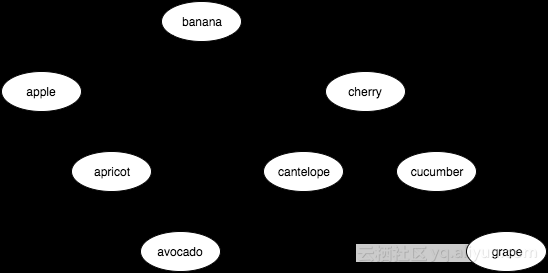// 按顺序遍历
BinaryTree.forEach = function forEach(visitFn,node){
if (node) {
if (node.left) {
forEach( visitFn, node.left );
}

visitFn( node );

if (node.right) {
forEach( visitFn, node.right );
}
}
};

BinaryTree.forEach( node => console.log( node.value ), banana );
// apple apricot avocado banana cantelope cherry cucumber grape

// 访问 cherry 作为根的子树
BinaryTree.forEach( node => console.log( node.value ), cherry );
// cantelope cherry cucumber grape

BinaryTree.map = function map(mapperFn,node){
if (node) {
let newNode = mapperFn( node );
newNode.parent = node.parent;
newNode.left = node.left ?
map( mapperFn, node.left ) : undefined;
newNode.right = node.right ?
map( mapperFn, node.right ): undefined;

if (newNode.left) {
newNode.left.parent = newNode;
}
if (newNode.right) {
newNode.right.parent = newNode;
}

return newNode;
}
};

var BANANA = BinaryTree.map(
node => BinaryTree( node.value.toUpperCase() ),
banana
);

BinaryTree.forEach( node => console.log( node.value ), BANANA );
// APPLE APRICOT AVOCADO BANANA CANTELOPE CHERRY CUCUMBER GRAPE

BANANA 是一个与 banana 不同的树（所有节点都不同），就像在一个数组上调用 map(..) 会返回一个新数组一样。正如其他对象/数组的数组一样，如果 node.value 本身引用了一些对象/数组，那么如果你想要更深层的不可变性的话，你还需要在映射函数中手动拷贝它。

BinaryTree.reduce = function reduce(reducerFn,initialValue,node){
if (arguments.length < 3) {
// 更换参数，因为 initialValue 被省略了
node = initialValue;
}

if (node) {
let result;

if (arguments.length < 3) {
if (node.left) {
result = reduce( reducerFn, node.left );
}
else {
return node.right ?
reduce( reducerFn, node, node.right ) :
node;
}
}
else {
result = node.left ?
reduce( reducerFn, initialValue, node.left ) :
initialValue;
}

result = reducerFn( result, node );
result = node.right ?
reduce( reducerFn, result, node.right ) : result;
return result;
}

return initialValue;
};

BinaryTree.reduce(
(result,node) => result.concat( node.value ),
[],
banana
);
//   "cherry","cucumber","grape"]

BinaryTree.filter = function filter(predicateFn,node){
if (node) {
let newNode;
let newLeft = node.left ?
filter( predicateFn, node.left ) : undefined;
let newRight = node.right ?
filter( predicateFn, node.right ) : undefined;

if (predicateFn( node )) {
newNode = BinaryTree(
node.value,
node.parent,
newLeft,
newRight
);
if (newLeft) {
newLeft.parent = newNode;
}
if (newRight) {
newRight.parent = newNode;
}
}
else {
if (newLeft) {
if (newRight) {
newNode = BinaryTree(
undefined,
node.parent,
newLeft,
newRight
);
newLeft.parent = newRight.parent = newNode;

if (newRight.left) {
let minRightNode = newRight;
while (minRightNode.left) {
minRightNode = minRightNode.left;
}

newNode.value = minRightNode.value;

if (minRightNode.right) {
minRightNode.parent.left =
minRightNode.right;
minRightNode.right.parent =
minRightNode.parent;
}
else {
minRightNode.parent.left = undefined;
}

minRightNode.right =
minRightNode.parent = undefined;
}
else {
newNode.value = newRight.value;
newNode.right = newRight.right;
if (newRight.right) {
newRight.right.parent = newNode;
}
}
}
else {
return newLeft;
}
}
else {
return newRight;
}
}

return newNode;
}
};

var vegetables = [ "asparagus", "avocado", "brocolli", "carrot",
"celery", "corn", "cucumber", "lettuce", "potato", "squash",
"zucchini" ];

// 过滤作物的列表，使之仅含蔬菜
node => vegetables.indexOf( node.value ) != -1,
banana
);

// 购物单
BinaryTree.reduce(
(result,node) => result.concat( node.value ),
[],
);
// ["avocado","cucumber"]

## 总结

• map(..)：将值投射到新列表中时将其变形。
• filter(..)：将值投射到新列表中时选择或排除它。
• reduce(..)：将一个列表中的值结合为另一个值（通常但不总是数组）。OC中 多参数省略函数的声明和实现
<div style="color:rgb(70,70,70); font-family:simsun; font-size:14px; line-height:21px; text-indent:2em"> <span style="word-wrap:normal; word-break:normal">OC开发中，我们也会发现会有类似于NSLog（</span>NSString *
1674 01054 0[CareerCup] 13.9 Aligned Malloc and Free Function 写一对申请和释放内存函数
1017 0643 0966 0【案例】MySQL count操作优化案例一则
3316 0Java经典类库-Guava中的函数式编程讲解

809 0vb 操作窗体中控件的函数定义
title(i)   Public Sub AddDataForListView(form1 As Form, controlName As String)'窗体与窗体中要操作的控件名称  form1.Controls(controlName).属性... End Sub
572 0JavaScript立即调用的函数表达式

622 0JavaScript创建对象（四）——组合使用构造函数和原型模式

900 0

12

0

JS零基础入门教程（上册）